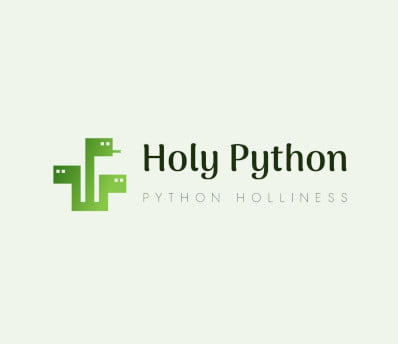# K-Means (Step by Step)

Here is a breakdown of a K-Means machine learning implementation.

K-Means is the most popular unsupervised algorithm that is used for clustering.

Although it only clusters spherical shapes and can’t deal with arbitrarily shaped clusters K-Means is very practical and relatively performant making it a commonly utilized solution for clustering unlabeled data.

K-Means assigns centroids to data and then aims to find optimum centroid points and cluster members relatively by minimizing the inertia (sum of distance between centroids and relative member points).

You can read about K-Means history and check out pros and cons of K-Means algorithms.

This Machine Learning tutorial elaborates the Simple K-Means Implementation and tries to lay out a very simple, easy to follow and easy to digest step by step explanation.

10 mins

#### Course Provider

Provided by HolyPython.comWe’ve split up K-Means implementation to 2 different sections here:

(Red for the actual machine learning work and black font signifies preparation phase)

1. Import the relevant Python libraries
2. Import the data
Create a train / test split
4. Create the K-Means model object
5. Fit the model
Predict
6. Evaluate the accuracy
Let’s read more about each individual step and what’s achieved with each of them:

## 1 Import Libraries

pandas can be useful for constructing dataframes and scikit learn is the ultimate library for simple machine learning operations and practicing machine learning.

This is the standard process for reading data. Reading data is simple but there can be important points such as: dealing with columns, headers, titles, constructing data frames etc.

## 4 Create the Model

Machine Learning models can be created with a very simple and straight-forward process using scikitlearn. In this case we will create a K-Means Clustering object from the K-Means module of scikitlearn.cluster library.

## -Predict

There is no prediction step either. As the model is fit with data centroids, clusters and inertia will be calculated.

## 2 Import the Data

We need a dataset that’s unlabeled and appropriate for unsupervised machine learning implementations via K-Means. Scikitlearn has such sample datasets but you can also make any dataset unlabeled by excluding the labels.

## -Split the Data

This step is skipped as there is no training in unsupervised machine learning techniques.

## 5 Fit the Model

K-Means also needs to be fit but this is rather introducing data to K-Means than an actual training process as there is no training in unsupervised machine learning implementations.

## 6 Evaluation

Evaluation of clustering methods is a bit different than classification or regression evaluations. Firstly there is no label so we don’t have a way of numerically evaluating the accuracy of results.

K-Means evaluation is usually done empirically based on expertise and past experience as well as cross validation techniques.

### 1- Importing the libraries

First the import part for libraries:

• sklearn.cluster provides the clustering model for K-Means
• datasets module of sklearn has great datasets making it easy to experiment with AI & Machine Learning
``````###Importing Libraries
from sklearn.cluster import KMeans
from sklearn import datasets``````

### 2- Importing the data (iris dataset)

Good old Iris dataset can be good idea since it is a familiar dataset and it would be interesting to see the results of clustering on this dataset.

``````###Importing Dataset

### 3- Reading the data (scikitlearn datasets and pandas dataframe)

In this case we don’t need to bother with constructing data frames either since we only need the unlabeled independent variables from the dataset which is already present in load_iris() module.

### 4- Creating the model (cluster.KMeans)

At this stage we can create the K-Means model. It’s also where we define some of the important hyperparameters of the model such as n_clusters and n_init. Here we are aiming to create 4 clusters in total and try the centroid initiation 12 times.

``````###Creating K-Means Clustering Model
k_means = KMeans(init = "k-means++", n_clusters = 4, n_init = 12)``````

You can see our K-Means optimization tutorial for a detailed introduction of K-Means Hyperparameter Optimization.

### 5- Fitting the model (Where clustering happens)

Training using the .fit method.

``````###Fitting the Model
k_means.fit(iris)
``````

### 6- Evaluating results

K-Means results can be evaluated by adjusting n_clusters parameter and based on domain expertise. Evaluating the results of clusters can be a subjective outcome since any number of clusters can be placed on a logical frame eventually. This is why expertise is critical when evaluating clustering results.

Another approach can be visualizing the cluster outcomes for a visual inspection as well as cross validation through the employment of various different clustering algorithms such as DBSCAN or Hierarchical Clustering models.

You can see the full one piece code in this page: K-Means Simple Implementation